# Homology of spheres

This article describes the value (and the process used to compute it) of some homotopy invariant(s) for a topological space or family of topological spaces. The invariant is homology group and the topological space/family is sphere
Get more specific information about sphere | Get more computations of homology group

In this article, we briefly describe the homology groups of spheres, a proof using the Mayer-Vietoris homology sequence, and explanations in terms of cellular and simplicial homology.

## Statement

### Reduced version over integers

For$n$ a nonnegative integer, we have the following result for the reduced homology groups:$\tilde{H}_k(S^n) = 0, k \ne n$:

and:$\tilde{H}_n(S^n) \cong \mathbb{Z}$

### Unreduced version over integers

We need to make cases based on whether$n = 0$ or$n$ is a positive integer:

•$n = 0$ case: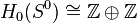$H_0(S^0) \cong \mathbb{Z} \oplus \mathbb{Z}$ and$H_k(S^0)$ is trivial for$k > 0$.
•$n > 0$ case:$H_0(S^n) \cong H_n(S^n) \cong \mathbb{Z}$ and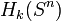$H_k(S^n)$ is trivial for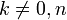$k \ne 0,n$.

### Reduced version over a module$M$ over a ring$R$

For$n$ a nonnegative integer, we have the following result for the reduced homology groups:$\tilde{H}_k(S^n) = 0, k \ne n$:

and: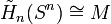$\tilde{H}_n(S^n) \cong M$

### Unreduced version over a module$M$ over a ring$R$

We need to make cases based on whether$n = 0$ or$n$ is a positive integer:

•$n = 0$ case: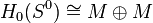$H_0(S^0) \cong M \oplus M$ and$H_k(S^0)$ is trivial for$k > 0$.
•$n > 0$ case: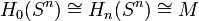$H_0(S^n) \cong H_n(S^n) \cong M$ and$H_k(S^n)$ is trivial for$k \ne 0,n$.

## Related invariants

These are all invariants that can be computed in terms of the homology groups.

Invariant General description Description of value for spheres
Betti numbers The$k^{th}$ Betti number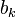$b_k$ is the rank of the$k^{th}$ homology group. For$n = 0$,$b_0 = 2$, all other$b_k$ are$0$; for$n > 0$,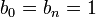$b_0 = b_n = 1$, all other$b_k$s are$0$.
Poincare polynomial Generating polynomial for Betti numbers$2$ for$n = 0$,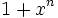$1 + x^n$ for$n > 0$
Euler characteristic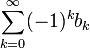$\sum_{k=0}^\infty (-1)^k b_k$$2$ for$n$ even,$0$ for$n$ odd.

## Proof using singular homology

### Equivalence of reduced and unreduced version

The equivalence follows from the fact that reduced and unreduced homology groups coincide for$k > 0$ and for$k = 0$, the unreduced homology group is obtained from the reduced one by adding a copy of$\mathbb{Z}$ (or, if working over another ring or module, the base ring or module).

### Proof of reduced version

The case$n = 0$ is clear: the space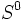$S^0$ is a discrete two-point space, hence it has two single-point path components, so the zeroth homology group is$M^{2 - 1} = M^1 = M$. Higher homology groups are trivial because the cycle and boundary groups both coincide with the group of all functions to$S^0$, so the homology group is trivial.

In general, we use induction, starting with the base case$n = 0$. The inductive step follows from fact (1) and the fact that each$S^n$ is the suspension of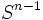$S^{n-1}$.

## Proof using cellular homology

See (2): CW structure of spheres.

## Proof using simplicial homology

See (3): Simplicial structure of spheres.